# Legendre symbol

(diff) ← Older revision | Latest revision (diff) | Newer revision → (diff)

An arithmetic function of the numbersand, defined for odd prime numbersand integersnot divisible by. The Legendre symbol is denoted by. The Legendre symbolif the congruence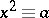is solvable; otherwise,. The Legendre symbol is sometimes defined for numbersdivisible byby puttingin this case. The Legendre symbol has the following properties:

1) if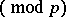, then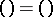;

2)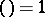;

3)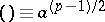;

4)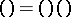;

5)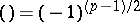;

6)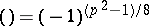;

7) ifandare odd prime numbers, then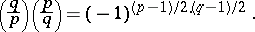The last fact, first proved by C.F. Gauss (1796), is called the quadratic reciprocity law. The above properties make it possible to calculate the Legendre symbol easily, without resorting to solving congruences. For example,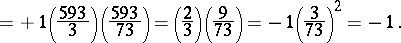The calculation of the Legendre symbol is facilitated still more by the use of the Jacobi symbol. For fixedthe Legendre symbol is a real character of the multiplicative group of residue classes modulo(cf. Character of a group).

It was introduced by A.M. Legendre in 1785.

How to Cite This Entry:
Legendre symbol. Encyclopedia of Mathematics. URL: http://encyclopediaofmath.org/index.php?title=Legendre_symbol&oldid=14735
This article was adapted from an original article by Yu.V. Nesterenko (originator), which appeared in Encyclopedia of Mathematics - ISBN 1402006098. See original article